# Position control exampleusing SimpleFOCMini

For this BLDC motor position control example we are going to be using this hardware:

Arduino UNOArduino SimpleFOCMiniAMT 103 encoderIPower GBM4108H-120T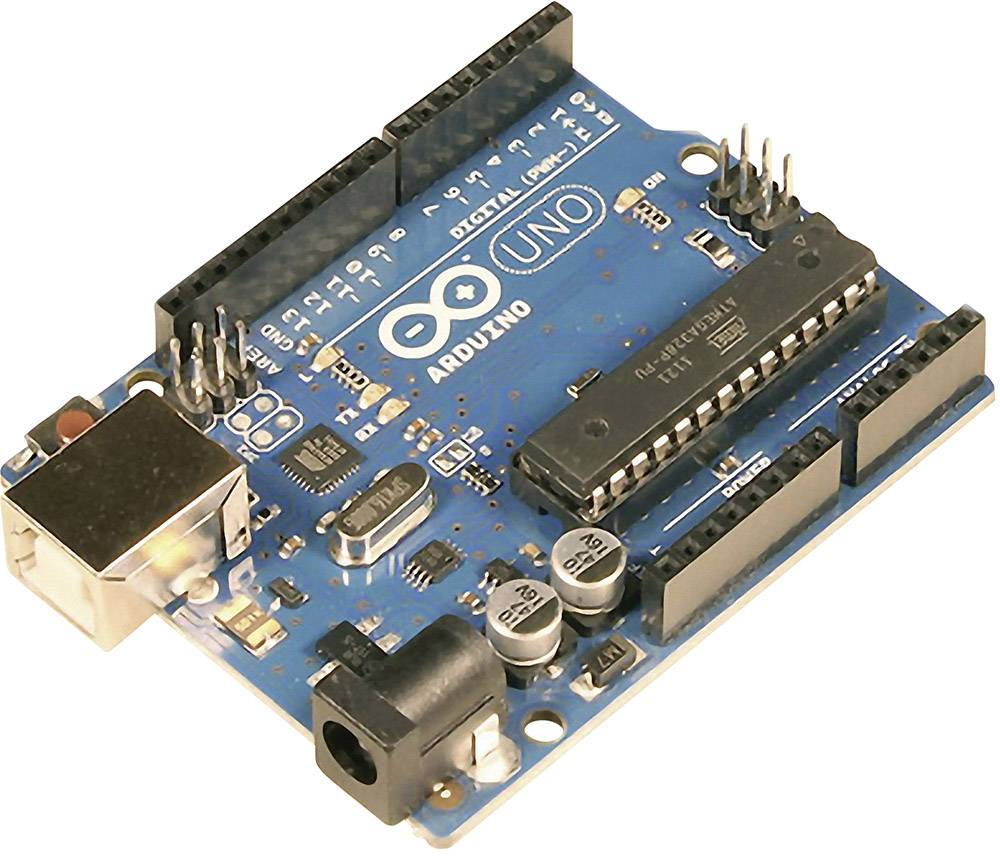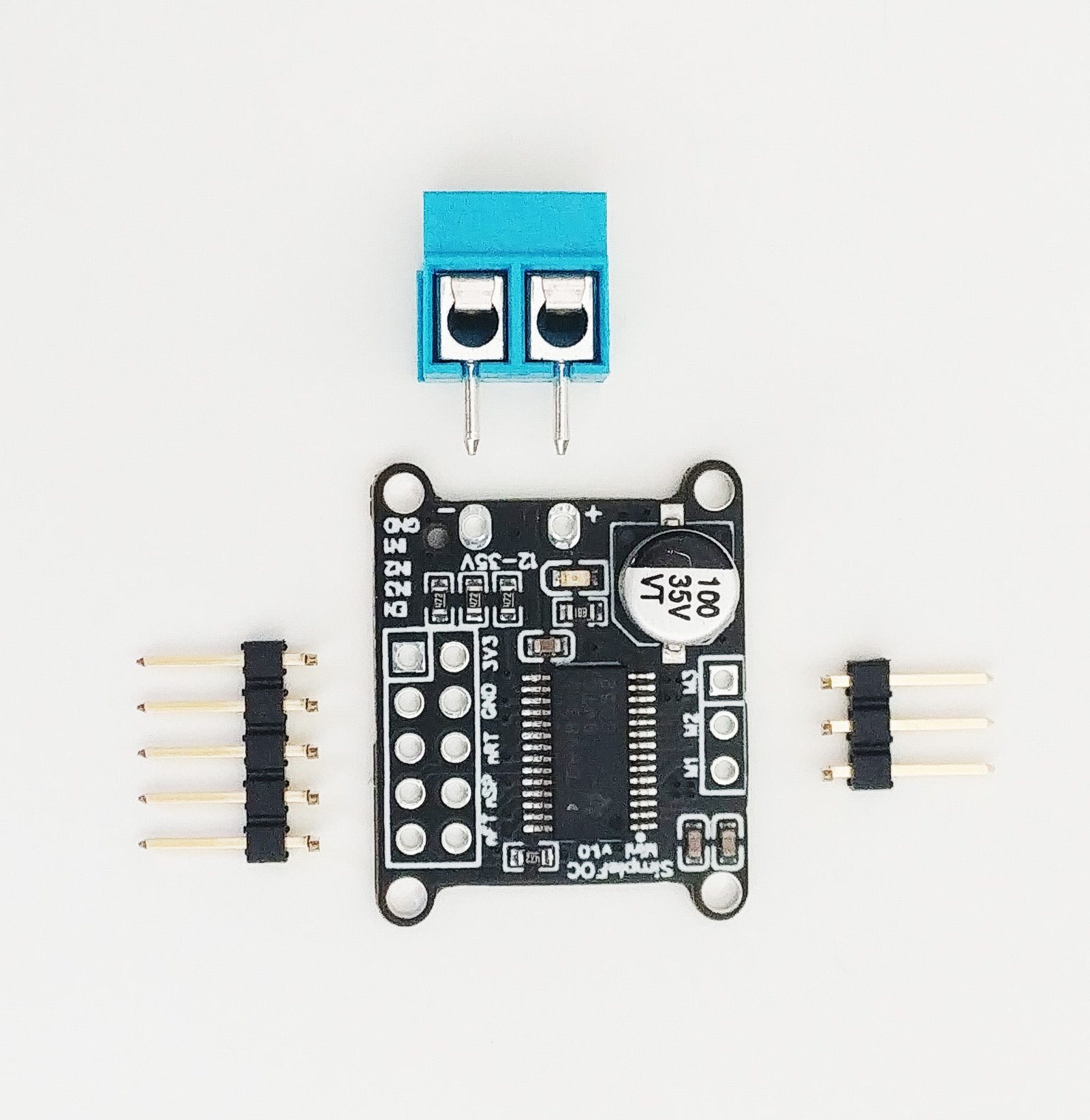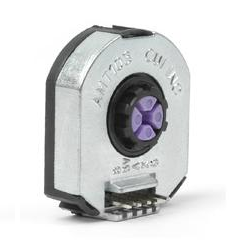# Connecting everything together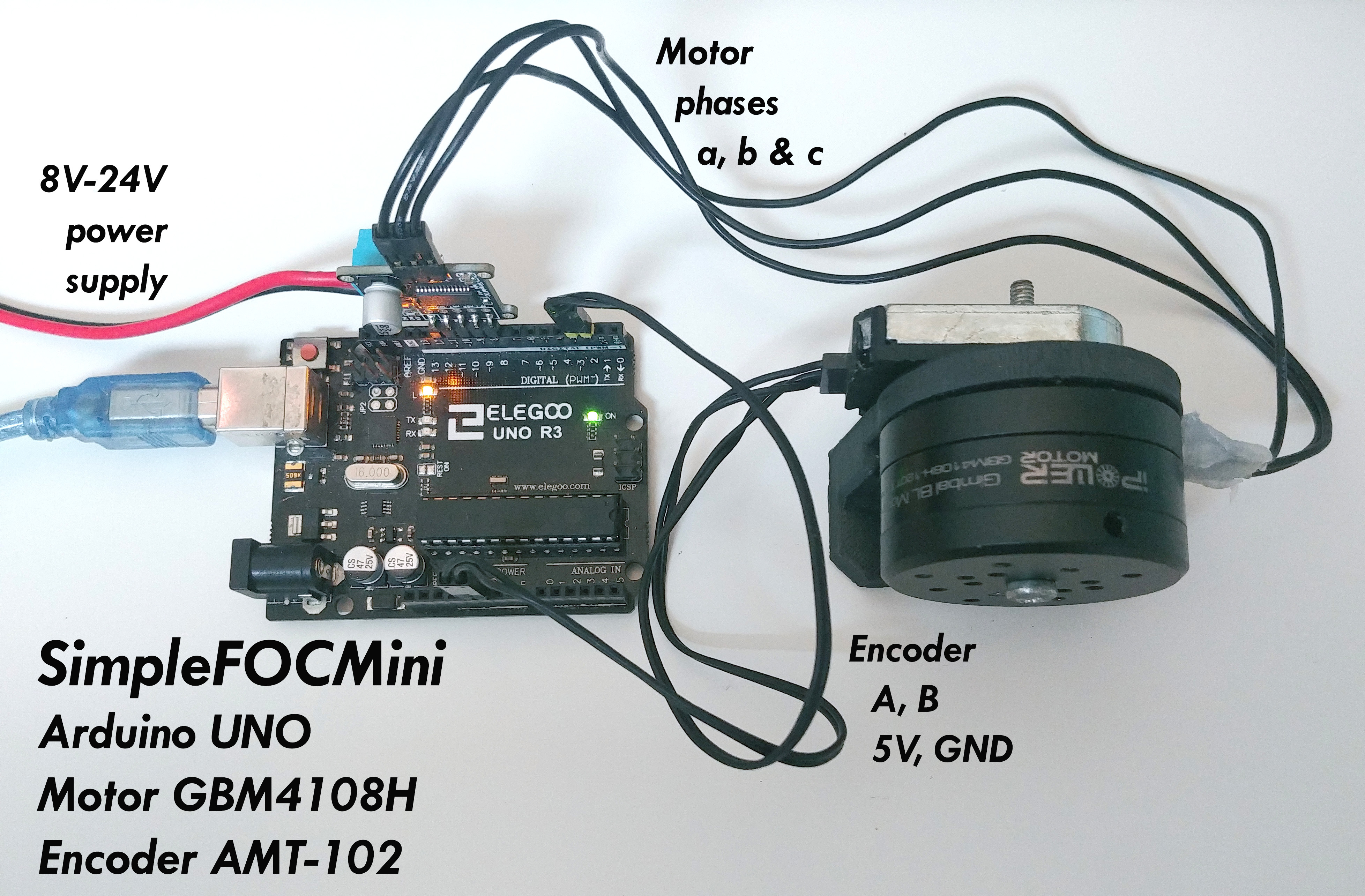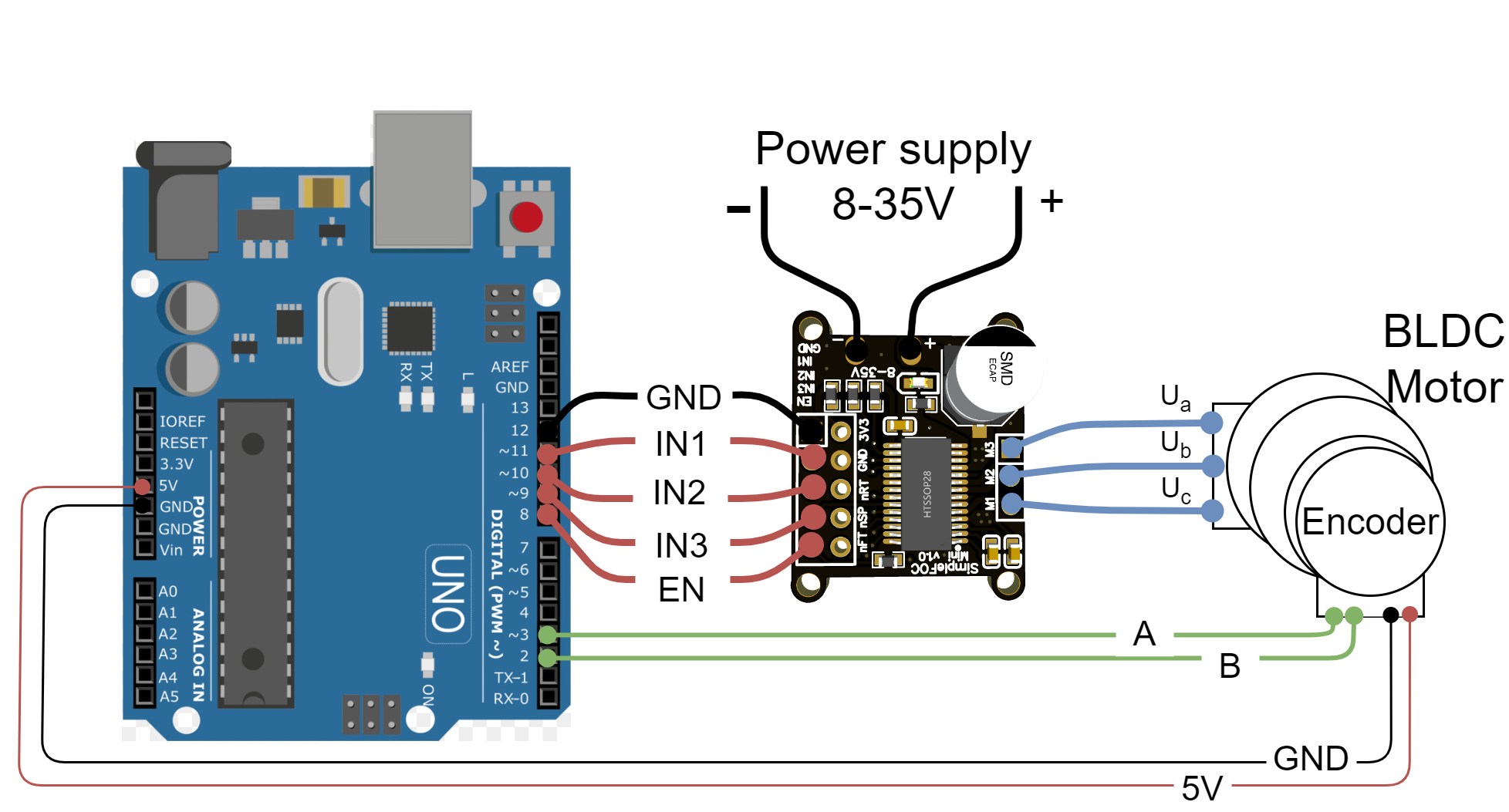## Encoder

• Channels A and B are connected to the Arduino UNO pins 2 and 3.

## Motor

• Motor phases a, b and c are connected directly the motor terminal connector connectors M1, M2 and M3 of the SimpleFOCMini board

## SimpleFOCMini

• The most convenient way of plugging the SimpleFOCMini board to the Arduino UNO is to stack it on its pins 12-8.
• GND - 12
• IN1 - 11
• IN2 - 10
• IN3 - 9
• EN - 8

Pin 12 is not a real ground pin. As no power is transferred through the mini’s GND pin then we can use pin 12 to approximate the GND pin by declaring it as digital output and setting it to LOW. This technique might not always work though. If you start seeing that your motor vibrates, even in the open loop control mode, then you should ditch this approach and connect the mini’s GND pin to the Arduino UNO’s GND pin. The vibrations should then stop completely.

### Small motivation :D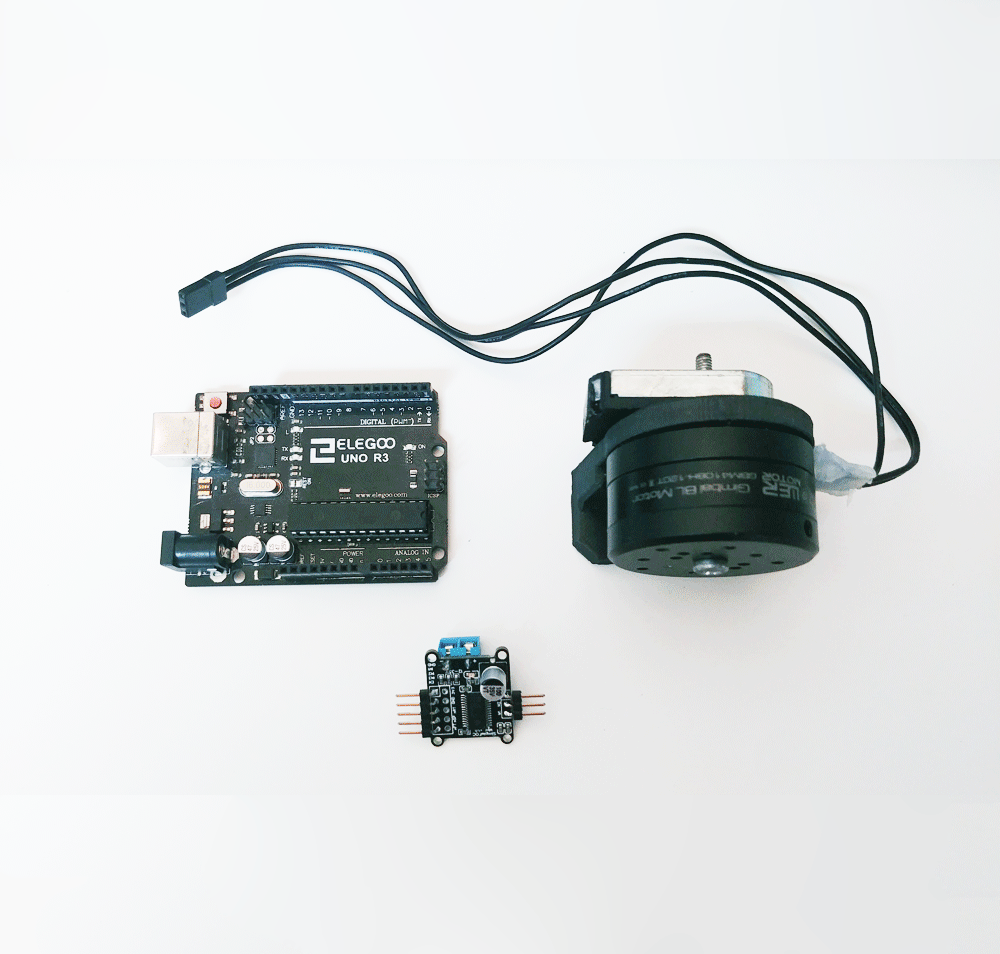# Arduino code

Let’s go through the full code for this example and write it together. First thing you need to do is include the SimpleFOC library:

#include <SimpleFOC.h>


Make sure you have the library installed. If you still don’t have it please check the get started page

## Encoder code

First we define the Encoder class with the A and B channel pins and number of impulses per revolution.

// define Encoder
Encoder encoder = Encoder(2, 3, 2048);


Then we define the buffering callback functions.

// channel A and B callbacks
void doA(){encoder.handleA();}
void doB(){encoder.handleB();}


In the setup() function we initialize the encoder and enable interrupts:

// initialize encoder hardware
encoder.init();
// hardware interrupt enable
encoder.enableInterrupts(doA, doB);


And that is it, let’s setup the motor.

For more configuration parameters of the encoders please check the Encoder class docs.

## Motor code

First we need to define the BLDCMotor class with the number od pole pairs (11)

// define BLDC motor
BLDCMotor motor = BLDCMotor(11);

If you are not sure what your pole pairs number is please check the find_pole_pairs.ino example.

Next we need to define the BLDCDriver3PWM class with the PWM pin numbers of the motor and the driver enable pin

// define BLDC driver
BLDCDriver3PWM driver = BLDCDriver3PWM(11, 10, 9, 8);


Then in the setup() we configure first the voltage of the power supply if it is not 12 Volts and init the driver.

// power supply voltage
// default 12V
driver.voltage_power_supply = 12;
driver.init();


Additionally we add the code to declare the pin 12 ad digital output set to LOW to be used as a common ground signal. In the setup we add

pinMode(12,OUTPUT); // declares pin 12 as output and sets it to LOW


Then we tell the motor which control loop to run by specifying the motor.controller variable.

// set control loop type to be used
// MotionControlType::torque
// MotionControlType::velocity
// MotionControlType::angle
motor.controller = MotionControlType::angle;


Now we configure the velocity PI controller parameters

// velocity PI controller parameters
// default P=0.5 I = 10
motor.PID_velocity.P = 0.2;
motor.PID_velocity.I = 20;

//default voltage_power_supply
motor.voltage_limit = 6;


Additionally we can configure the Low pass filter time constant Tf

// velocity low pass filtering
// default 5ms - try different values to see what is the best.
// the lower the less filtered
motor.LPF_velocity.Tf = 0.02;


Finally we configure position P controller gain and the velocity limit variable.

// angle P controller
// default P=20
motor.P_angle.P = 20;
//  maximal velocity of the position control
// default 20
motor.velocity_limit = 4;


Next we connect the encoder and the driver to the motor, do the hardware init and init of the Field Oriented Control.

// link the motor to the sensor
// link the motor to the driver

// initialize motor
motor.init();
// align encoder and start FOC
motor.initFOC();


The last peace of code important for the motor is of course the FOC routine in the loop function.

void loop() {
// iterative FOC function
motor.loopFOC();

// iterative function setting and calculating the angle/position loop
// this function can be run at much lower frequency than loopFOC function
motor.move();
}


That is it, let’s see the full code now!

For more configuration parameters and control loops please check the BLDCMotor class doc.

## Full Arduino code

To the full code I have added a small serial commander interface, to be able to change position/angle target value in real time.

#include <SimpleFOC.h>

// init BLDC motor
BLDCMotor motor = BLDCMotor( 11 );
// init driver
BLDCDriver3PWM driver = BLDCDriver3PWM(11, 10, 9, 8);
//  init encoder
Encoder encoder = Encoder(2, 3, 2048);
// channel A and B callbacks
void doA(){encoder.handleA();}
void doB(){encoder.handleB();}

// commander interface
Commander command = Commander(Serial);
void onTarget(char* cmd){ command.motion(&motor, cmd); }

void setup() {

pinMode(12,OUTPUT); // declares pin 12 as output and sets it to LOW

// initialize encoder hardware
encoder.init();
// hardware interrupt enable
encoder.enableInterrupts(doA, doB);
// link the motor to the sensor

// power supply voltage
// default 12V
driver.voltage_power_supply = 12;
driver.init();
// link the motor to the driver

// set control loop to be used
motor.controller = MotionControlType::angle;

// controller configuration based on the control type
// velocity PI controller parameters
// default P=0.5 I = 10
motor.PID_velocity.P = 0.2;
motor.PID_velocity.I = 20;

//default voltage_power_supply
motor.voltage_limit = 6;

// velocity low pass filtering
// default 5ms - try different values to see what is the best.
// the lower the less filtered
motor.LPF_velocity.Tf = 0.02;

// angle P controller
// default P=20
motor.P_angle.P = 20;
//  maximal velocity of the position control
// default 20
motor.velocity_limit = 4;

// initialize motor
motor.init();
// align encoder and start FOC
motor.initFOC();

// monitoring port
Serial.begin(115200);
Serial.println("Set the target angle using serial terminal:");
_delay(1000);
}

void loop() {
// iterative FOC function
motor.loopFOC();

// function calculating the outer position loop and setting the target position
motor.move();

// commander interface with the user
commander.run();

}